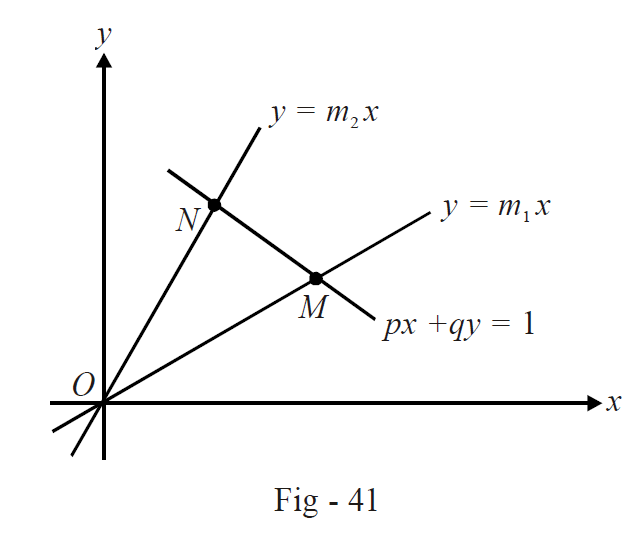# Straight Lines Set 4

Go back to  'Straight Lines'

Example – 7

The curves

\begin{align} {C_1}:{a_1}{x^2} + 2{h_1}xy + {b_1}{y^2} + 2{g_1}x = 0 \\ {C_2}:{a_2}{x^2} + 2{h_2}xy + {b_2}{y^2} + 2{g_2}x = 0 \\ \end{align}

intersect at two points A and B other than the origin. Find the condition for OA and OB to be perpendicular.

Solution: Assume the equation of AB to be  $$y = mx + c.$$ Thus, using the homogenizing technique, we can write the joint equation of OA and OB:

Homogenizing C1:

\begin{align}&{a_1}{x^2} + 2{h_1}xy + {b_1}{y^2} + 2{g_1}x\left( {\frac{{y - mx}}{c}} \right) = 0 \\ \Rightarrow \qquad &\left( {{a_1} - \frac{{2m{g_1}}}{c}} \right){x^2} + 2{h_1}xy + {b_1}{y^2} + \frac{{2{g_1}xy}}{c} = 0 \\ \end{align}

This is the joint equation of OA and OB. OA and OB are perpendicular if

\begin{align}&{a_1} - \frac{{2m{g_1}}}{c} + {b_1} = 0 \\ \Rightarrow \qquad &\frac{m}{c} = \frac{{{a_1} + {b_1}}}{{2{g_1}}}\qquad\qquad\qquad\qquad\dots(1) \\ \end{align}

Homogenizing C2: Similarly, we can again evaluate the joint equation of OA and OB by homogenizing the equation of $${C_2}:$$

$\left( {{a_2} - \frac{{2m{g_2}}}{c}} \right){x^2} + 2{h_2}xy + {b_2}{y^2} + \frac{{2{g_2}xy}}{c} = 0$

The perpendicularity condition gives

\begin{align} &{a_2} - \frac{{2m{g_2}}}{c} + {b_2} = 0 \\ \Rightarrow \qquad &\frac{m}{c} = \frac{{{a_2} + {b_2}}}{{2{g_2}}}\,\,\,\,\,\,\,\,\,\,\,\,\,\,\,\,\,\,\,\,\,\,\,\,\,\,\,\,\,\,\,\,\,\,\,\, \ldots \left( 2 \right) \\ \end{align}

From (1) and (2), the necessary required condition is

$\frac{{{a_1} + {b_1}}}{{{g_1}}} = \frac{{{a_2} + {b_2}}}{{{g_2}}}$

Example – 8

Find the orthocentre of the triangle formed by the lines  $$a{x^2} + 2hxy + b{y^2} = 0$$ and $$px + qy = 1.$$

Solution: The two lines given by the joint equation pass through the origin. Assume their slopes to be m1 and m2 so that m1 and m2 are the roots of

\begin{align}& b{m^2} + 2hm + a = 0 \\ \Rightarrow\qquad &{m_1} + {m_2} = - \frac{{2h}}{b}, \;\;{m_1}{m_2} = \frac{a}{b}\,\,\,\,\,\,\,\,\,\,\,\,\,\,\,\,\,\,\,\,\,\,\,\,\,\,\,\,\,\,\,\,\,\,\,\,\,\,\, \ldots \left( 1 \right)\\ \end{align}To evaluate the orthocentre, we need two altitudes. We take one of them to be the one dropped from O onto MN.

$qx - py = 0\,\,\,\,\,\,\,\,\,\,\,\,\,\,\,\,\,\,\,\,\,\,\,\,\,\,\, \ldots \left( 2 \right)$

Let us now find the altitude from M onto ON. The coordinates of M are, by solving $$y = {m_1}x$$ and  $$px + qy = 1$$ simultaneously,

$M \equiv \left( {\frac{1}{{p + q{m_1}}},\,\,\frac{{{m_1}}}{{p + q{m_1}}}} \right)$

The slope of ON is m2 so that the slope of the altitude through M is  $$- \frac{1}{{{m_2}}};$$  thus, its equation is

\begin{align}&y - \frac{{{m_1}}}{{p + q{m_1}}} = \frac{{ - 1}}{{{m_2}}}\left( {x - \frac{1}{{p + q{m_1}}}} \right) \\ \Rightarrow \qquad& \frac{x}{{{m_2}}} + y = \frac{{1 + {m_1}{m_2}}}{{{m_2}(p + q{m_1})}} \\ \Rightarrow \qquad& x + {m_2}y = \frac{{1 + {m_1}{m_2}}}{{p + q{m_1}}}\,\,\,\,\,\,\,\,\,\,\,\,\,\,\,\,\,\,\,\,\,\,\,\,\,\,\,\,\,\,\,\,\,\,\,\,\,\,\,\,\,\,\,\,\,\,\, \ldots \left( 3 \right) \\ \end{align}

The intersection of (2) and (3) yields the orthocentre

\begin{align}h& = \frac{{p(1 + {m_1}{m_2})}}{{(p + q{m_1})(p + q{m_2})}} \\ &= \frac{{p(1 + {m_1}{m_2})}}{{{p^2} + pq({m_1} + {m_2}) + {q^2}{m_1}{m_2}}} \\ &= \frac{{p(a + b)}}{{b{p^2} - 2hpq + a{q^2}}} \\ k &= \frac{q}{p}.h\,\,\,\,\,\,\,\,\,\,\,\,\,\,\,\,\,\,\,\,\,\,\,\,\,\,\,\,\,\,\,\,\,\,\,\left( {from\left( 1 \right)} \right) \\& = \frac{{q(a + b)}}{{b{p^2} - 2hpq + a{q^2}}} \\ \end{align}

Thus, the orthocentre has the coordinates

$\left( {\frac{{p(a + b)}}{{b{p^2} - 2hpq + a{q^2}}},\,\,\,\frac{{q(a + b)}}{{b{p^2} - 2hpq + a{q^2}}}} \right)$